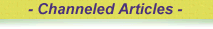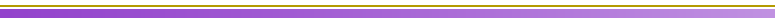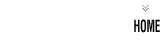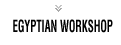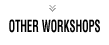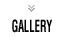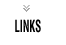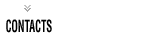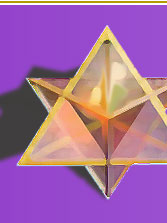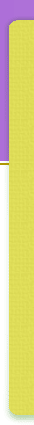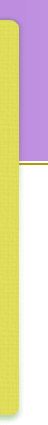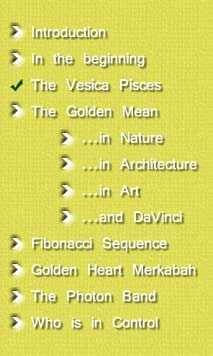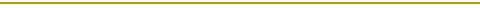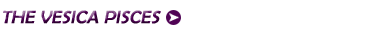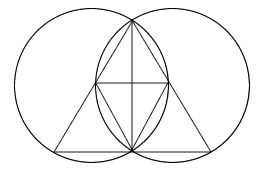When the I AM merges with I AM THAT I AM, the circumference of each circle passes through the centre of the opposite circle. It is the creation of a mirror image by Divinity to relate to itself which is the basis of a deep esoteric truth. All mirrors are a reflection of this!!! The shape which is formed by the coming together of the two circles is the Vesica Pisces. In the Pythagorean school it was discovered that the square roots of the numbers 2, 3, & 5 are fundamental properties that are inherent in the creation of all form. These square roots are to be found innate within the structure of the Vesica Pisces.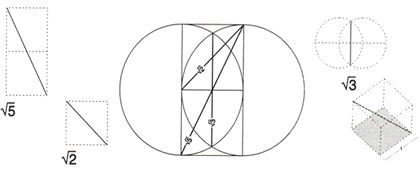The square root of 2 defines the diagonal of the square. Its quality is generation. 2 Squared = 1.4142135. The square root of 3 defines both the diagonal of a cube and the length of the Vesica Pisces. Its quality is the formation of 3-dimensional space. 3 Squared = 1.7320508. The square root of 5 defines the Golden Rectangle and the Golden Mean proportion. Its quality is regeneration or growth. It is seen to represent the life-giving qualities of Christ Energy. 5 Squared = 2.2360679.